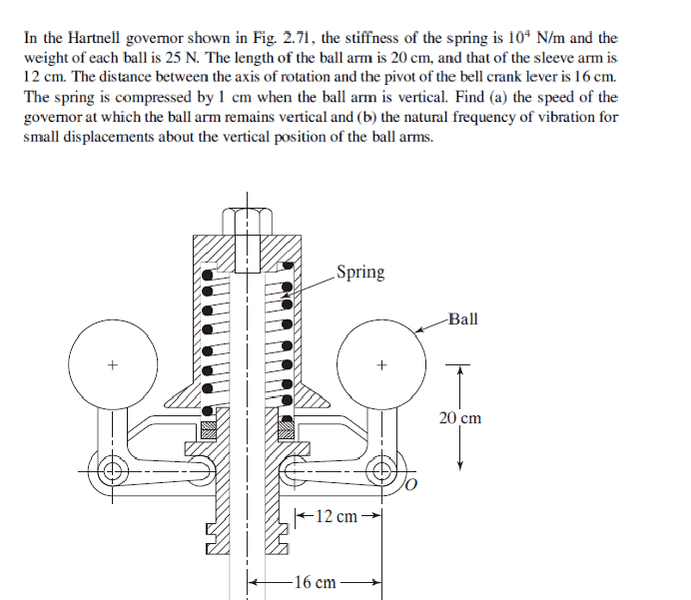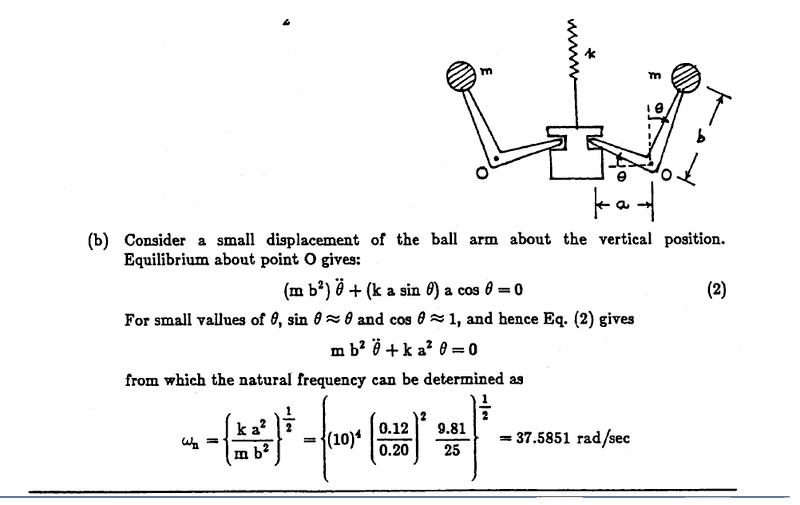# Determination of the natural frequency of a Hartnell governor

## Homework Statement## The Attempt at a Solution

I found this solution for the nature frequencybut here it does not include the Ball weight and centrifugal force in the moment balance equation about the pivot (O), it is wrong answer...is not it?

I tried to solve the problem this way, moment balance about pivot (O)
$$(mb^2)θ'' = -1/2*k*(1/100+a sinθ)a conθ + mgbsinθ + mrw^2bcosθ$$

where :
$$-1/2*k*(1/100+a sinθ)a conθ$$ : is the moment of the spring force
$$mgbsinθ$$: is the moment of the weight of the ball.
$$mrw2bcosθ$$: is the moment of the centrifugal force.
$$a=12cm,b=20cm,k=104N/m,mg=25N$$

for small displacment $$sinθ=θ,cosθ=1$$. then
$$(mb^2)θ′′=−1/2k(1/100+aθ)a+mgbθ+mrw^2b$$

r is the distance between ball center and the center of rotation.
$$r=(16/100+bsinθ)=(16/100+bθ)$$

the equation becomes
$$(mb^2)θ′′=−1/2∗k(1/100+aθ)a+mgbθ+m(16/100+bθ)w^2b$$
rearrangement of the equation
$$(m∗b^2)θ′′+(1/2∗ka^2+−mgb−mb^2w^2)θ+1/2∗k∗1/100∗a−m∗16/100∗w^2b=0$$
from this equation we could say that the nature frequency is
$$(w_n)^2=(1/2∗ka^2−mgb−mb^2w^2)/(mb^2)$$
which shows that the nature frequency changes with rotation speed. Is this a right solution?

Spinnor
Gold Member
Did you have to do part a of the problem? If so what did you get.

Spinnor
Gold Member
I think the last two equations are correct. In addition if you set θ and θ'' to zero you can solve for ω the angular velocity at equilibrium. I would not worry about the answers dependence on angular velocity. You look like you got the right answer, good work! Ask your teacher about your question.

Edit, remember ω for your problem is fixed.

Thank you for your reply and check of the solution. and when you set θ and θ'' to zero you would get the answer to part (a).